Publications are Open
Access in this journal
Article Versions
Export Article
• Normal Style
• MLA Style
• APA Style
• Chicago Style
Research Article
Open Access Peer-reviewed

Hroncová Darina , Miková Ľubica
American Journal of Mechanical Engineering. 2017, 5(6), 355-361. DOI: 10.12691/ajme-5-6-25
Published online: December 15, 2017

### Abstract

We present a kinematic analysis and computer simulation of a two-link mobile manipulator model. Mobility of the manipulator is achieved by an undercarriage. Its work space is defined by the upper part consisting of two rotationally connected arms attached to the carriage by a rotational bonding. The model is created and the simulation is carried out in MSC Adams. As a result of the analysis are the moments in the individual kinematic pairs at the given movement of the carriage of the mobile manipulator.

### 1. Introduction

The prototype testing, key to development and innovation processes, is by development of computer software largely replaced by computer simulations. These became an alternative to creating and operational testing of a physical prototype. This is motivated with an effort to increase the design quality before the prototype is built. Simulation also shortens the time of prototype design.

There are different developers offering simulation software for dynamic simulation of mechanical systems. Some of them are Solid Works, Matlab, Ansys, MSC Nastran, MSC Adams.

Users who want to simulate complex dynamics of mechanical systems, including elastic members, MSC Adams package is suitable. The user can simulate the behavior of the model obtaining its dynamic and kinematic characteristics.

### 2. Simulation Model

Looking at a mobile robot from a kinematic point of view, it can be divided into two mechanical systems, namely the moving carriage and the upper part (Figure 1). The carriage allows expansion of the working area of the robot. The upper part with the end effector is used to perform the required action.

• Figure 1. Model of the mobile manipulator

The upper part of the manipulator model in the picture (Figure 2) is composed of two members with lengths l1 and l2, which are connected to each other by a rotational kinematic pair and with a second rotational kinematic pair to the carriage 1. The respective weights of the manipulator arms are m1 and m2.

• Figure 2. Upper part of the manipulator

Parameters Θ1 and Θ2 indicate the angles of rotation of respective arm.

Kinematic equations describing the position of the end point M as a function of the angles of rotation Θ1 and Θ2 are expressed in the form 2: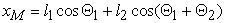(1)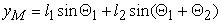(2)

The driving moments M1 a M2 are generally delivered by servomotors in individual kinematic pairs. They determine the movement of the mechanism (Figure 2). Motion equations are derived by using Lagrange equations of the second kind. They can by written in the following matrix notation 3, 4, 5, 6: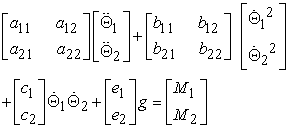(3)

where 6: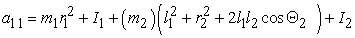(4)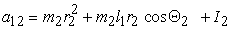(5)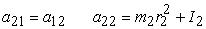(6)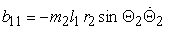(7)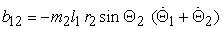(8)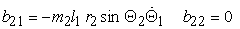(9)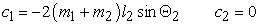(10)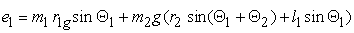(11)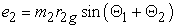(12)

Equation (3) represents the system of two differential equations of the second order. Lagrange's equations of motion did not consider the mechanical inaccuracy and the effect of friction in individual kinematic pairs, nor did they consider the elastic deformations of the manipulator's arms 1.

### 3. Computer Simulation

The aim was to create a 3D model of the manipulator using modeling elements and procedures for creating body, geometric, kinematic links in the MSC Adams/View environment and to verify its functionality after the model compilation 7, 8, 9. The assembled model of the manipulator is shown in Figure 3.

• Figure 3. Scheme of the manipulator

The model of the manipulator in different views is shown in Figure 4.

The interactive simulation and visualization allows a comfortable connection between the simulation process and modification of model parameters and visualization of the effect of these modifications. The charts of quantities of interest are shown in windows and give the user the ability to check their current values along with ongoing simulation (Figure 5).

Postprocessing as the presentation tool is an integral part of the process of simulation of the virtual prototype (Figure 6).

• Figure 4. Different views of the model
• Figure 5. Charts of torques in the joints of the manipulator
• Figure 6. MSC Adams postprocessor

An extremely comfortable tool for creating, processing, modifying and presenting simulation results in graphs 10, 11, 12 has been incorporated into MSC Adams (Figure 7, Figure 8).

• Figure 7. MSC Adams postprocessor
• Figure 8. MSC Adams postprocessor

### 4. Simulation Results

The values of parameters in graphic form, obtained in postprocessor are provided in the next pictures (Figure 9-Figure 11).

• Figure 9. The graph of the Torque τ1 of the joint dependent on time
• Figure 10. The graph of the Torque τ2 of the joint dependent on time

We also obtained values of kinematics quantities displacement, velocity and acceleration of the end effector point M (Figure 12 - Figure 17).

• Figure 11. The graph of the SFORCE_1 of the joint in the end – effector dependent on time
• Figure 12. The graph of the displacement xM of the end-effector dependent on time
• Figure 13. The graph of the displacement yM of the end-effector dependent on time
• Figure 14. The graph of the velocity vxM of the end-effector dependent on time
• Figure 15. The graph of the velocity vyM of the end-effector dependent on time
• Figure 16. The graph of the acceleration axM of the end-effector dependent on time
• Figure 17. The graph of the acceleration ayM of the end-effector dependent on time

In the next picture (Figure 18) the trajectory of the end point M is drawn.

• Figure 18. The graph of the trajectory of the end-effector

### 5. Conclusion

At present, we encounter the need to deploy mobile robots. The paper is a demonstration of simulation and possibilities of using computer simulation software in the kinematic analysis of such composite mobile robot systems. An important advantage of computer simulation of prototype models is the possibility to instantly visualize the variations and modifications of the design as well as their consequences to the behavior of the whole system. Displaying the complete system in motion in real time simultaneously verifying and reporting potential collisions of individual elements and displaying the quantities of interest is another benefit of computer simulation. Achieved realism of animation is remarkable, so it is not surprising that it leads to a better understanding of the system and a more successful verification of the construction.

### Acknowledgements

This work was supported by grant projects VEGA 1/0872/16 Research of synthetic and biological inspired locomotion of mechatronic systems in rugged terrain.

### References

  Bocko, J., Frankovský, P., Delyová, I., Pástor, M., Kinematika v príkladoch, SjF TU v Košiciach, 2011. In article  Juliš, K., Brepta, R., Mechanika II.díl, Dynamika, SNTL, Praha, 1987. In article  Craig J.J., Introduction to robotics: mechanics and control. Upper Saddle River: Pearson Prentice Hall, 2005. In article View Article  Murray R.M., Li Z. and Sastry S.S. A Mathematical Introduction to Robotic Manipulation. University of California: CRC Press, 1994. In article View Article  Lewis F.L., Dawson D.M. and Abdallah Ch. T., Robot Manipulator Control, Theory and Practice. New York: Marcel Dekker, USA, 2004. In article View Article  Paul, R.P., Robot manipulators, Mathematics, Programming and Control, Cambridge, MA: MIT Press, 1981. In article View Article  Ostertagová, E., “Modelling using polynomial regression,” Modelling of Mechanical and Mechatronics Systems 2012, November 6th-8th 2012, Zemplínska Šírava, Slovakia, In: Procedia Engineering, Vol. 48 (2012), p. 500-506. In article View Article  Virgala, I., Frankovský, P., Kenderová, M., “Friction Effect Analysis of a DC Motor,” In: American Journal of Mechanical Engineering, Vol. 1, No. 1, p. 1-5, 2013. In article View Article  Hroncová, D., Frankovský, P., Bettes, G., “Kinematical Analysis of Crank Slider Mechanism with Graphical Method and by Computer Simulation” In: American Journal of Mechanical Engineering, Vol. 4, No. 7, p. 329-343, 2016. In article View Article  Šarga, P., Hroncová, D., Rákay, R., “The MSC Adams/View and Simulation of the Crank Rocker Mechanism,” In: American Journal of Mechanical Engineering, Vol. 3, No. 6, p. 161-164, 2015. In article View Article  Hroncová, D., Šarga, P., “Kinematics analysis of the crank mechanism conveyor Using MSC Adams,” In: Applied Mechanics and Materials, Trans Tech Publications, Switzerland, Vol. 816, p. 140-149, 2015. In article View Article  Miková, Ľ., Gmiterko, A., Hroncová, D., “State Space Representation of Dynamical Systems,” In: American Journal of Mechanical Engineering, Vol. 4, No. 7, 385-389, 2016. In article View ArticleThis work is licensed under a Creative Commons Attribution 4.0 International License. To view a copy of this license, visit http://creativecommons.org/licenses/by/4.0/